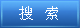说明：双击或选中下面任意单词，将显示该词的音标、读音、翻译等；选中中文或多个词，将显示翻译。 您的位置：首页 -> 句库 -> 算术及逻辑运算 1. arithmetic and logic unit data flow算术及逻辑运算部件数据流 2. The arithmetic logic unit (ALU), which performs arithmetic and logical operations.算术逻辑单元，用来进行算术逻辑运算。 3. arithmetic logic register stack (ALRS)算术及逻辑寄存器栈 4. To perform the operation of negation.实现逻辑“非”运算。 5. 3.1.6 Logical operators3.1.6 逻辑运算符 6. On the Mathematical Logic Operation "→" and BCK Operation "*;关于逻辑运算“→”和BCK运算“*” 7. The logical operation which makes use of the AND operator or logical product.使用AND运算符或逻辑乘积的逻辑运算。 8. calculator with arithmetic logic带有算术逻辑的计算器 9. arithmetic logic of pocket calculator袖珍计算机的算术逻辑 10. A Design of YHFT s Multiplier Unit and ALU;“银河飞腾”DSP乘法部件及算术逻辑运算部件的设计 11. A term sometimes used synonymously with" arithmetic(logic) unit" .本术语有时用作“运算（逻辑）器”的同义语。 12. Data processing--Vocabulary--Section 02: Arithmetic and logic operationsGB/T5271.2-1988数据处理词汇02部分算术和逻辑运算 13. Proposition relativity and logic calculation in probabilistic logic;概率逻辑中的命题相关性与逻辑运算 14. register arithmetic logic unit寄存器运算逻辑部件 15. Properties of Temporal Logic Operators and Extended Operations on Intuitionistic Fuzzy Singular Rough Sets 直觉模糊S-粗集时态逻辑算子及扩展运算性质 16. ALU：Arithmetic Logic Unit; the circuitry within the CPU which performs all arithmetic functions. 算术逻辑运算器：执行运算功能的中央处理器内的线路。 17. An operation table for a logic operation.用于逻辑运算的一种运算表。 18. Division Operation Semantics and Realization by SQL Based on Predicate Logic;基于谓词逻辑的除法运算语义及其SQL实现 ©2011 dictall.com